## How to solve exponential equations

Examples of exponential equations.

$$2^{\red x} = 4 \\ 8^{\red{2x}} = 16 \\ \\ 16^{\red { x+1}} = 256 \\ \left( \frac{1}{2} \right)^{\red { x+1}} = 512$$

As you might've noticed, an exponential equation is just a special type of equation. It's an equation that has exponents that are $$\red{ variables}$$.

## Steps to Solve

There are different kinds of exponential equations. We will focus on exponential equations that have a single term on both sides. These equations can be classified into 2 types.

$$4^x = 4^9$$.

$$4^3 = 2^x$$.

$$\left( \frac{1}{4} \right)^x = 32$$ (Part II below)

## Part I. Solving Exponential Equations with Same Base

Solve: $$4^{x+1} = 4^9$$

Ignore the bases, and simply set the exponents equal to each other

$$x + 1 = 9$$

Solve for the variable

$$x = 9 - 1 \\ x = \fbox { 8 }$$

$$4^{x+1} = 4^9 \\ 4^{\red{8}+1} = 4^9$$ $$4^{\red{9} } = 4^9$$

## Exponential Equation Solver

Enter any exponential equation into the algebra solver below :

## II. Solving Exponential Equations with un -like bases

What do they look like.

$$\red 4^3 = \red 2^x$$ $$\red 9^x = \red { 81 }$$ $$\left( \red{\frac{1}{2}} \right)^{ x+1} = \red 4^3$$ $$\red 4^{2x} +1 = \red { 65 }$$

In each of these equations, the base is different. Our goal will be to rewrite both sides of the equation so that the base is the same.

Solve: $$4^{3} = 2^x$$Answer : They are both powers of 2

Rewrite equation so that both exponential expressions use the same base

$$\red 4^{3} = 2^x \\ (\red {2^2})^{3} = 2^x$$

Use exponents laws to simplify

$$(\red {2^2})^{3} = 2^x \\ (2^\red {2 \cdot 3 }) = 2^x \\ (2^\red 6 ) = 2^x$$

Solve like an exponential equation of like bases

$$(2^\red 6 ) = 2^x \\ x = \fbox{6}$$

Substitute $$\red 6$$ into the original equation to verify our work.

$$4^{3} = 2^{\red 6}$$ $$64 = 64$$

## Example with Negative Exponent

Unlike bases often involve negative or fractional bases like the example below. We are going to treat these problems like any other exponential equation with different bases--by converting the bases to be the same.

## Practice Problems ( un -like bases)

Solve the following exponential Equation: $$9^x = 81$$You can use either 3 or 9 . I will use 9.

$\\ 81 = \red 9 ^{\blue 2} \\ 9 = \red 9 ^{\blue 1} \\$

Substitute the rewritten bases into original equation

$$(\red 9^{\blue 1})^x = \red 9^{\blue 2}$$ 2 ) x =3 4 -->

$$(\red 9^{\blue 1})^x = \red 9^{\blue 2} \\ 9^{1 \cdot x } = 9 ^{2} \\ 9^{x } = 9 ^{2}$$ 2 x =3 4 -->

$$x = 2$$ -->

Solve the equation : $$4^{2x} +1 = 65$$

Rewrite this equation so that it looks like the other ones we solved. Isolate the exponential expression as follows:

$$4^{2x} +1 \red{-1} = 65\red{-1} \\ 4^{2x} = 64$$They are both powers of 2 and of 4 . You could use either base to solve this. I will use base 4

$\\ 64 = \red 4 ^{\blue 3} \\ 4 = \red 4 ^{\blue 1} \\$

$$4^{2x} = 64 \\ \red 4^{\blue{ 2x }} = \red 4^{\blue 3 }$$

$$\red 4^{\blue{ 2x }} = \red 4^{\blue 3 }$$ 2x = 4 3 -->

Not much to do this time :)

$$2x = 3 \\ x = \frac{3}{2}$$

Solve the exponential Equation : $$\left( \frac{1}{4} \right)^x = 32$$

Since these equations have different bases, follow the steps for unlike basesThey are both powers of 2

$\\ 32 = \red 2 ^{\blue 5} \\ \frac 1 4 = \red 2 ^{\blue {-2}} \\$

Rewrite as a negative exponent and substitute the rewritten bases into original equation

$$\left( \frac{1}{4} \right)^x = 32 \\ \left( \frac{1}{2^2} \right)^x = 32 \\ \left(\red 2 ^{\blue{-2}} \right)^x = \red 2^{\blue 5}$$

$$\left(\red 2 ^{\blue{-2}} \right)^x = \red 2^{\blue 5} \\ 2 ^{-2 \cdot x} = 2^5 \\ 2 ^{-2x} = 2^5$$

$$2 ^{-2x} = 2^5 \\ -2x = 5 \\ \frac{-2x}{-2} = \frac{5}{-2} \\ x = -\frac{5}{2}$$

Solve this exponential equation: $$\left( \frac{1}{9} \right)^x-3 = 24$$

$$\left( \frac{1}{9} \right)^x -3 \red{+3} =24\red{+3} \\ \left( \frac{1}{9} \right)^x=27$$They are both powers of 3 .

$\\ \frac 1 9 = \red 3 ^{\blue {-2}} \\ 27 = \red 3 ^{\blue 3} \\$

Rewrite as a negative exponent and substitute into original equation

$$\left( \frac{1}{9} \right)^x=27 \\ \left( \red{3^{-2}}\right)^x=\red{3^3 }$$

$$3^\red{{-2 \cdot x}} = 3^3 \\ 3^\red{{-2x}} = 3^3$$

$$-2x = 3 \\ x = \frac{3}{-2} \\ x = -\frac{3}{2}$$

Solve this exponential equation: $$\left( \frac{1}{25} \right)^{(3x -4)} -1 = 124$$

Rewrite this equation so that it looks like the other ones we solved--In other words, isolate the exponential expression as follows:

$$\left( \frac{1}{25} \right)^{(3x -4)} -1 \red{+1} = 124 \red{+1} \\ \left( \frac{1}{25} \right)^{(3x -4)} = 125$$They are both powers of 5 .

$\\ \frac { 1 } { 25 } = \red 5 ^{\blue {-2}} \\ 125 = \red 5 ^{\blue 3} \\$

$$\left( \frac{1}{25} \right)^{(3x -4)} = 125 \\ \left( \red{5^{-2}} \right)^{(3x -4)} = \red{5^3}$$

$$5^\red{{-2 \cdot (3x -4)}} = 5^3 \\ 5^\red{{(-6x + 8)}} = 5^3$$

$$-6x + 8 =3 \\ -6x = -5 \\ x = \frac{-5}{-6} \\ x = \frac{5}{6}$$

• Laws of Exponents
• Rules of Exponents

## Ultimate Math Solver (Free) Free Algebra Solver ... type anything in there!

Popular pages @ mathwarehouse.com.## Exponential Equations

Exponential equations , as the name suggests, involve exponents. We know that the exponent of a number (base) indicates the number of times the number (base) is multiplied. But, what happens if the power of a number is a variable? When the power is a variable and if it is a part of an equation, then it is called an exponential equation. We may need to use the connection between the exponents and logarithms to solve the exponential equations.

Let us learn the definition of exponential equations along with the process of solving them when the bases are the same and when the bases are not the same along with a few solved examples and practice questions.

## What are Exponential Equations?

An exponential equation is an equation with exponents where the exponent (or) a part of the exponent is a variable . For example, 3 x = 81, 5 x - 3 = 625, 6 2y - 7 = 121, etc are some examples of exponential equations. We may come across the use of exponential equations when we are solving the problems of algebra, compound interest , exponential growth , exponential decay , etc.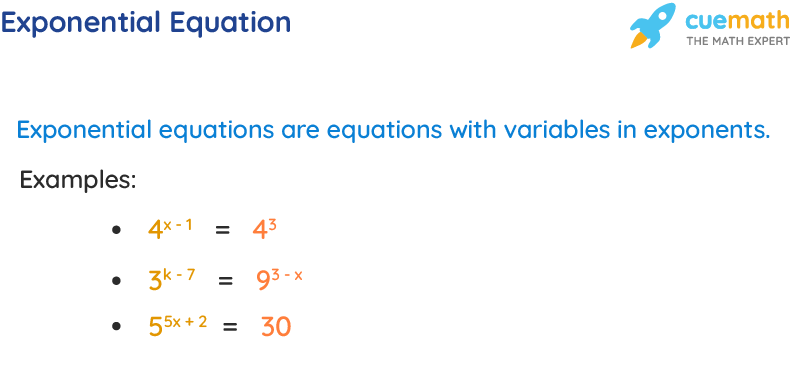## Types of Exponential Equations

There are three types of exponential equations. They are as follows:

• Equations with the same bases on both sides. (Example: 4 x = 4 2 )
• Equations with different bases that can be made the same. (Example: 4 x = 16 which can be written as 4 x = 4 2 )
• Equations with different bases that cannot be made the same. (Example: 4 x = 15)

## Equations with Exponents

The equations in algebra involving variable exponents are called equations with exponents or exponential equations. In other words, we can say that algebraic equations in which variables occur as exponents are known as the equations with exponents. Some of the examples of such an equation are, 3 x + 4  = 81, -2 3y-7  = -64, etc.

## Exponential Equations Formulas

While solving an exponential equation , the bases on both sides may be the same or may not be the same. Here are the formulas that are used in each of these cases, which we will learn in detail in the upcoming sections.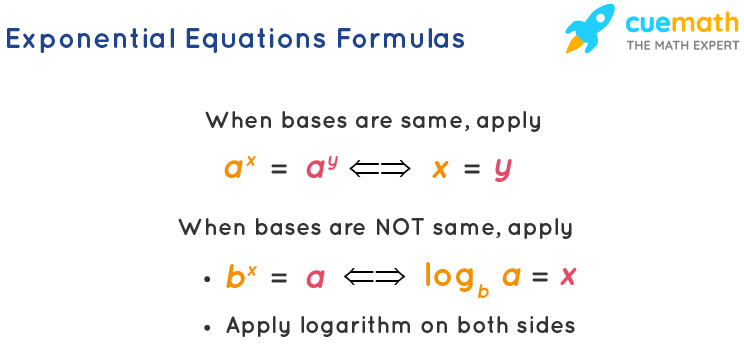## Property of Equality for Exponential Equations

This property is useful to solve an exponential equation with the same bases. It says when the bases on both sides of an exponential equation are equal, then the exponents must also be equal. i.e.,

a x = a y ⇔ x = y.

## Exponential Equations to Logarithmic Form

We know that logarithms are nothing but exponents and vice versa. Hence an exponential equation can be converted into a logarithmic function . This helps in the process of solving an exponential equation with different bases. Here is the formula to convert exponential equations into logarithmic equations.

b x = a ⇔ log b a = x

## Solving Exponential Equations With Same Bases

Sometimes, an exponential equation may have the same bases on both sides of the equation. For example, 5 x = 5 3 has the same base 5 on both sides. Sometimes, though the exponents on both sides are not the same, they can be made the same. For example, 5 x = 125. Though it doesn't have the same bases on both sides of the equation, they can be made the same by writing it as 5 x = 5 3 (as 125 = 5 3 ). To solve the exponential equations in each of these cases, we just apply the property of equality of exponential equations, using which, we set the exponents to be the same and solve for the variable.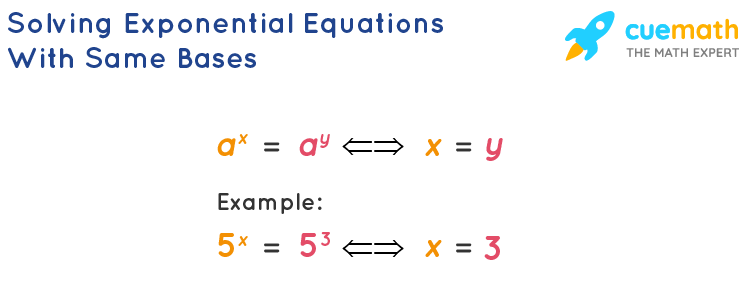Here is another example where the bases are not the same but can be made the same.

Example: Solve the exponential equation 7 y + 1 = 343 y .

We know that 343 = 7 3 . Using this, the given equation can be written as,

7 y + 1 = (7 3 ) y

7 y + 1 = 7 3y

Now the bases on both sides are the same. So we can set the exponents to be the same.

Subtracting y from both sides,

Dividing both sides by 2,

## Solving Exponential Equations With Different Bases

Sometimes, the bases on both sides of an exponential equation may not be the same (or) cannot be made the same. We solve the exponential equations using logarithms when the bases are not the same on both sides of the equation. For example, 5 x = 3 neither has the same bases on both sides nor the bases can be made the same. In such cases, we can do one of the following things.

• Convert the exponential equation into the logarithmic form using the formula b x = a ⇔ log b a = x and solve for the variable.
• Apply logarithm (log) on both sides of the equation and solve for the variable. In this case, we will have to use a property of logarithm , log a m = m log a.

We will solve the equation 5 x = 3 in each of these methods.

We will convert 5 x = 3 into logarithmic form. Then we get,

log 5 3 = x

Using the change of base property ,

x = (log 3) / (log 5)

We will apply log on both sides of 5 x = 3. Then we get, log 5 x = log 3. Using the property log a m = m log a on the left side of the equation, we get, x log 5 = log 3. Dividing both sides by log 5,

Important Notes on Exponential Equations:

Here are some important notes with respect to the exponential equations.

• To solve the exponential equations of the same bases, just set the exponents equal.
• To solve the exponential equations of different bases, apply logarithm on both sides.
• The exponential equations with the same bases also can be solved using logarithms.
• If an exponential equation has 1 on any one side, then we can write it as 1 = a 0 , for any 'a'. For example, to solve 5 x = 1, we can write it as 5 x = 5 0 , then we get x = 0.
• To solve an exponential equation using logarithms, we can either apply "log" or apply "ln" on both sides.

Related Articles:

• Exponential Form
• Exponent Rules
• Exponential Functions
• Exponential Equation Calculator

## Exponential Equations Examples

Example 1: Solve 27 / (3 -x ) = 3 6 .

We know that 27 = 3 3 . We can make the bases to be the same on both sides using this.

3 3 / (3 -x ) = 3 6

Using the quotient property of exponents, a m /a n = a m - n . Using this,

3 3 - (-x) = 3 6

3 3 + x = 3 6

Now the bases on both sides are the same. So we can set the exponents to be equal.

Subtracting 3 from both sides,

Therefore, the solution of the given exponential equation is x = 3.

Example 2: Solve the exponential equation 7 3x + 7 = 490.

490 cannot be written as a power of 7. So we cannot make the bases to be the same here. So we solve this exponential equation using logarithms.

Apply log on both sides of the given equation,

log 7 3x + 7 = log 490

Using a property of logarithms , log a m = m log a. Using this,

(3x + 7) log 7 = log 490 ... (1)

Here, 490 = 49 × 10 = 7 2 × 10.

So, log 490 = log (7 2 × 10)

= log 7 2 + log 10 (because log (mn) = log m + log n)

= 2 log 7 + 1 (because log a m = m log a and log 10 = 1)

Substituting this in (1),

(3x + 7) log 7 = 2 log 7 + 1

Dividing both sides by log 7,

3x + 7 = (2 log 7 + 1) / (log 7)

3x + 7 = 2 + (1 / log 7)

Subtracting 7 from both sides,

3x = -5 + (1 / log 7)

Dividing both sides by 3,

x = -5/3 + (1 / (3 log 7))

Answer: The solution of the given exponential equation is x = -5/3 + (1 / (3 log 7)).

Example 3: How long does it take for $20,000 to double if the amount is compounded annually at 8% annual interest? Round your answer to the nearest integer. The principal amount, P =$20000.

The rate of interest is, r = 8% = 8/100 = 0.08.

The final amount is, A = 20000 x 2 = $40,000 Let us assume that the required time in years is t. Using the compound interest formula when compounded annually, A = P (1 + r) t 40000 = 20000 (1 + 0.08) t Dividing both sides by 20000, 2 = (1.08) t Taking log on both sides, log 2 = log (1.08) t log 2 = t log (1.08) t = (log 2) / (log 1.08) The final answer is rounded to the nearest integer. Answer: It takes 9 years for$20,000 to double itself.

go to slide go to slide go to slideBook a Free Trial Class

## Exponential Equations Questions

go to slide go to slide

## FAQs on Exponential Equations

What are exponential equations.

An exponential equation is an equation that has a variable in its exponent(s). For example, 5 2x - 3 = 125, 3 7 - 2x = 91, etc are exponential equations.

## What Are Types of Exponential Equations?

There are three types of exponential equations. They are,

• The exponential equations with the same bases on both sides.
• The exponential equations with different bases on both sides that can be made the same.
• The exponential equations with different bases on both sides that cannot be made the same.

## How To Solve Exponential Equations?

To solve the exponential equations of equal bases, we set the exponents equal whereas to solve the exponential equations of different bases, we apply logarithms on both sides.

## How To Write Exponential Equation in Logarithmic Form?

Writing the exponential equation in the logarithmic form helps us to solve it. This can be done using the formula b x = a ⇔ log b a = x.

## What Is the Property of Equality of Exponential Equations?

The equality property of exponential equations says to set the exponents equal whenever the bases on both sides of the equation are equal. i.e., a x = a y ⇔ x = y.

## How To Solve Exponential Equations of Same bases?

When an exponential equation has the same bases on both sides, just set the exponents equal and solve for the variable. Here is an example, 4 2x - 1 = 4 1 - x . Here the bases on both sides are equal. So we can set the exponents equal. 2x - 1 = 1 - x 3x = 2 x = 2/3.

## How To Solve Exponential Equations of Different bases?

When an exponential equation has different bases on both sides, apply log on both sides and solve for the variable. Here is an example, 4 x - 5 = 8. Taking log on both sides, log 4 x - 5 = log 8 (x - 5) log 4 = log 8 x - 5 = (log 8) / (log 4) x = [(log 8) / (log 4)] + 5.

## How To Solve Exponential Equations Using Logarithms?

We solve exponential equations using logarithms in two ways.

• Convert the exponential equation into logarithmic equation using b x = a ⇔ log b a = x.
• Apply "log" or "ln" on both sides and solve.
• 6.6 Exponential and Logarithmic Equations
• Introduction to Prerequisites
• 1.1 Real Numbers: Algebra Essentials
• 1.2 Exponents and Scientific Notation
• 1.3 Radicals and Rational Exponents
• 1.4 Polynomials
• 1.5 Factoring Polynomials
• 1.6 Rational Expressions
• Key Equations
• Key Concepts
• Review Exercises
• Practice Test
• Introduction to Equations and Inequalities
• 2.1 The Rectangular Coordinate Systems and Graphs
• 2.2 Linear Equations in One Variable
• 2.3 Models and Applications
• 2.4 Complex Numbers
• 2.6 Other Types of Equations
• 2.7 Linear Inequalities and Absolute Value Inequalities
• Introduction to Functions
• 3.1 Functions and Function Notation
• 3.2 Domain and Range
• 3.3 Rates of Change and Behavior of Graphs
• 3.4 Composition of Functions
• 3.5 Transformation of Functions
• 3.6 Absolute Value Functions
• 3.7 Inverse Functions
• Introduction to Linear Functions
• 4.1 Linear Functions
• 4.2 Modeling with Linear Functions
• 4.3 Fitting Linear Models to Data
• Introduction to Polynomial and Rational Functions
• 5.2 Power Functions and Polynomial Functions
• 5.3 Graphs of Polynomial Functions
• 5.4 Dividing Polynomials
• 5.5 Zeros of Polynomial Functions
• 5.6 Rational Functions
• 5.7 Inverses and Radical Functions
• 5.8 Modeling Using Variation
• Introduction to Exponential and Logarithmic Functions
• 6.1 Exponential Functions
• 6.2 Graphs of Exponential Functions
• 6.3 Logarithmic Functions
• 6.4 Graphs of Logarithmic Functions
• 6.5 Logarithmic Properties
• 6.7 Exponential and Logarithmic Models
• 6.8 Fitting Exponential Models to Data
• Introduction to Systems of Equations and Inequalities
• 7.1 Systems of Linear Equations: Two Variables
• 7.2 Systems of Linear Equations: Three Variables
• 7.3 Systems of Nonlinear Equations and Inequalities: Two Variables
• 7.4 Partial Fractions
• 7.5 Matrices and Matrix Operations
• 7.6 Solving Systems with Gaussian Elimination
• 7.7 Solving Systems with Inverses
• 7.8 Solving Systems with Cramer's Rule
• Introduction to Analytic Geometry
• 8.1 The Ellipse
• 8.2 The Hyperbola
• 8.3 The Parabola
• 8.4 Rotation of Axes
• 8.5 Conic Sections in Polar Coordinates
• Introduction to Sequences, Probability and Counting Theory
• 9.1 Sequences and Their Notations
• 9.2 Arithmetic Sequences
• 9.3 Geometric Sequences
• 9.4 Series and Their Notations
• 9.5 Counting Principles
• 9.6 Binomial Theorem
• 9.7 Probability

## Learning Objectives

In this section, you will:

• Use like bases to solve exponential equations.
• Use logarithms to solve exponential equations.
• Use the definition of a logarithm to solve logarithmic equations.
• Use the one-to-one property of logarithms to solve logarithmic equations.
• Solve applied problems involving exponential and logarithmic equations.In 1859, an Australian landowner named Thomas Austin released 24 rabbits into the wild for hunting. Because Australia had few predators and ample food, the rabbit population exploded. In fewer than ten years, the rabbit population numbered in the millions.

Uncontrolled population growth, as in the wild rabbits in Australia, can be modeled with exponential functions. Equations resulting from those exponential functions can be solved to analyze and make predictions about exponential growth. In this section, we will learn techniques for solving exponential functions.

## Using Like Bases to Solve Exponential Equations

The first technique involves two functions with like bases. Recall that the one-to-one property of exponential functions tells us that, for any real numbers b , b , S , S , and T , T , where b > 0 , b ≠ 1 , b > 0 , b ≠ 1 , b S = b T b S = b T if and only if S = T . S = T .

In other words, when an exponential equation has the same base on each side, the exponents must be equal. This also applies when the exponents are algebraic expressions. Therefore, we can solve many exponential equations by using the rules of exponents to rewrite each side as a power with the same base. Then, we use the fact that exponential functions are one-to-one to set the exponents equal to one another, and solve for the unknown.

For example, consider the equation 3 4 x − 7 = 3 2 x 3 . 3 4 x − 7 = 3 2 x 3 . To solve for x , x , we use the division property of exponents to rewrite the right side so that both sides have the common base, 3. 3. Then we apply the one-to-one property of exponents by setting the exponents equal to one another and solving for x x :

## Using the One-to-One Property of Exponential Functions to Solve Exponential Equations

For any algebraic expressions S and  T , S and  T , and any positive real number b ≠ 1 , b ≠ 1 ,

Given an exponential equation with the form b S = b T , b S = b T , where S S and T T are algebraic expressions with an unknown, solve for the unknown.

• Use the rules of exponents to simplify, if necessary, so that the resulting equation has the form b S = b T . b S = b T .
• Use the one-to-one property to set the exponents equal.
• Solve the resulting equation, S = T , S = T , for the unknown.

## Solving an Exponential Equation with a Common Base

Solve 2 x − 1 = 2 2 x − 4 . 2 x − 1 = 2 2 x − 4 .

Solve 5 2 x = 5 3 x + 2 . 5 2 x = 5 3 x + 2 .

## Rewriting Equations So All Powers Have the Same Base

Sometimes the common base for an exponential equation is not explicitly shown. In these cases, we simply rewrite the terms in the equation as powers with a common base, and solve using the one-to-one property.

For example, consider the equation 256 = 4 x − 5 . 256 = 4 x − 5 . We can rewrite both sides of this equation as a power of 2. 2. Then we apply the rules of exponents, along with the one-to-one property, to solve for x : x :

Given an exponential equation with unlike bases, use the one-to-one property to solve it.

• Rewrite each side in the equation as a power with a common base.

## Solving Equations by Rewriting Them to Have a Common Base

Solve 8 x + 2 = 16 x + 1 . 8 x + 2 = 16 x + 1 .

Solve 5 2 x = 25 3 x + 2 . 5 2 x = 25 3 x + 2 .

## Solving Equations by Rewriting Roots with Fractional Exponents to Have a Common Base

Solve 2 5 x = 2 . 2 5 x = 2 .

Solve 5 x = 5 . 5 x = 5 .

Do all exponential equations have a solution? If not, how can we tell if there is a solution during the problem-solving process?

No. Recall that the range of an exponential function is always positive. While solving the equation, we may obtain an expression that is undefined.

## Solving an Equation with Positive and Negative Powers

Solve 3 x + 1 = −2. 3 x + 1 = −2.

This equation has no solution. There is no real value of x x that will make the equation a true statement because any power of a positive number is positive.

Figure 2 shows that the two graphs do not cross so the left side is never equal to the right side. Thus the equation has no solution.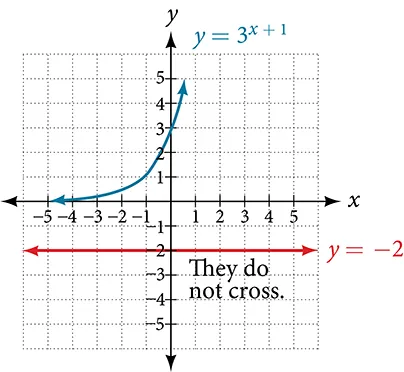Solve 2 x = −100. 2 x = −100.

## Solving Exponential Equations Using Logarithms

Sometimes the terms of an exponential equation cannot be rewritten with a common base. In these cases, we solve by taking the logarithm of each side. Recall, since log ( a ) = log ( b ) log ( a ) = log ( b ) is equivalent to a = b , a = b , we may apply logarithms with the same base on both sides of an exponential equation.

Given an exponential equation in which a common base cannot be found, solve for the unknown.

• If one of the terms in the equation has base 10, use the common logarithm.
• If none of the terms in the equation has base 10, use the natural logarithm.
• Use the rules of logarithms to solve for the unknown.

## Solving an Equation Containing Powers of Different Bases

Solve 5 x + 2 = 4 x . 5 x + 2 = 4 x .

Solve 2 x = 3 x + 1 . 2 x = 3 x + 1 .

Is there any way to solve 2 x = 3 x ? 2 x = 3 x ?

Yes. The solution is 0. 0.

## Equations Containing e

One common type of exponential equations are those with base e . e . This constant occurs again and again in nature, in mathematics, in science, in engineering, and in finance. When we have an equation with a base e e on either side, we can use the natural logarithm to solve it.

Given an equation of the form y = A e k t , y = A e k t , solve for t . t .

• Divide both sides of the equation by A . A .
• Apply the natural logarithm of both sides of the equation.
• Divide both sides of the equation by k . k .

## Solve an Equation of the Form y = Ae kt

Solve 100 = 20 e 2 t . 100 = 20 e 2 t .

Using laws of logs, we can also write this answer in the form t = ln 5 . t = ln 5 . If we want a decimal approximation of the answer, we use a calculator.

Solve 3 e 0.5 t = 11. 3 e 0.5 t = 11.

Does every equation of the form y = A e k t y = A e k t have a solution?

No. There is a solution when k ≠ 0 , k ≠ 0 , and when y y and A A are either both 0 or neither 0, and they have the same sign. An example of an equation with this form that has no solution is 2 = −3 e t . 2 = −3 e t .

## Solving an Equation That Can Be Simplified to the Form y = Ae kt

Solve 4 e 2 x + 5 = 12. 4 e 2 x + 5 = 12.

Solve 3 + e 2 t = 7 e 2 t . 3 + e 2 t = 7 e 2 t .

## Extraneous Solutions

Sometimes the methods used to solve an equation introduce an extraneous solution , which is a solution that is correct algebraically but does not satisfy the conditions of the original equation. One such situation arises in solving when the logarithm is taken on both sides of the equation. In such cases, remember that the argument of the logarithm must be positive. If the number we are evaluating in a logarithm function is negative, there is no output.

## Solving Exponential Functions in Quadratic Form

Solve e 2 x − e x = 56. e 2 x − e x = 56.

When we plan to use factoring to solve a problem, we always get zero on one side of the equation, because zero has the unique property that when a product is zero, one or both of the factors must be zero. We reject the equation e x = −7 e x = −7 because a positive number never equals a negative number. The solution ln ( −7 ) ln ( −7 ) is not a real number, and in the real number system this solution is rejected as an extraneous solution.

Solve e 2 x = e x + 2. e 2 x = e x + 2.

Does every logarithmic equation have a solution?

No. Keep in mind that we can only apply the logarithm to a positive number. Always check for extraneous solutions.

## Using the Definition of a Logarithm to Solve Logarithmic Equations

We have already seen that every logarithmic equation log b ( x ) = y log b ( x ) = y is equivalent to the exponential equation b y = x . b y = x . We can use this fact, along with the rules of logarithms, to solve logarithmic equations where the argument is an algebraic expression.

For example, consider the equation log 2 ( 2 ) + log 2 ( 3 x − 5 ) = 3.   log 2 ( 2 ) + log 2 ( 3 x − 5 ) = 3.   To solve this equation, we can use rules of logarithms to rewrite the left side in compact form and then apply the definition of logs to solve for x : x :

For any algebraic expression S S and real numbers b b and c , c , where b > 0 , b ≠ 1 , b > 0 , b ≠ 1 ,

## Using Algebra to Solve a Logarithmic Equation

Solve 2 ln x + 3 = 7. 2 ln x + 3 = 7.

Solve 6 + ln x = 10. 6 + ln x = 10.

## Using Algebra Before and After Using the Definition of the Natural Logarithm

Solve 2 ln ( 6 x ) = 7. 2 ln ( 6 x ) = 7.

Solve 2 ln ( x + 1 ) = 10. 2 ln ( x + 1 ) = 10.

## Using a Graph to Understand the Solution to a Logarithmic Equation

Solve ln x = 3. ln x = 3.

Figure 3 represents the graph of the equation. On the graph, the x -coordinate of the point at which the two graphs intersect is close to 20. In other words e 3 ≈ 20. e 3 ≈ 20. A calculator gives a better approximation: e 3 ≈ 20.0855. e 3 ≈ 20.0855.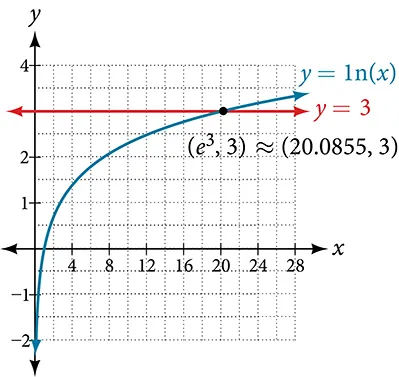Use a graphing calculator to estimate the approximate solution to the logarithmic equation 2 x = 1000 2 x = 1000 to 2 decimal places.## Using the One-to-One Property of Logarithms to Solve Logarithmic Equations

As with exponential equations, we can use the one-to-one property to solve logarithmic equations. The one-to-one property of logarithmic functions tells us that, for any real numbers x > 0 , x > 0 , S > 0 , S > 0 , T > 0 T > 0 and any positive real number b , b , where b ≠ 1 , b ≠ 1 ,

For example,

So, if x − 1 = 8 , x − 1 = 8 , then we can solve for x , x , and we get x = 9. x = 9. To check, we can substitute x = 9 x = 9 into the original equation: log 2 ( 9 − 1 ) = log 2 ( 8 ) = 3. log 2 ( 9 − 1 ) = log 2 ( 8 ) = 3. In other words, when a logarithmic equation has the same base on each side, the arguments must be equal. This also applies when the arguments are algebraic expressions. Therefore, when given an equation with logs of the same base on each side, we can use rules of logarithms to rewrite each side as a single logarithm. Then we use the fact that logarithmic functions are one-to-one to set the arguments equal to one another and solve for the unknown.

For example, consider the equation log ( 3 x − 2 ) − log ( 2 ) = log ( x + 4 ) . log ( 3 x − 2 ) − log ( 2 ) = log ( x + 4 ) . To solve this equation, we can use the rules of logarithms to rewrite the left side as a single logarithm, and then apply the one-to-one property to solve for x : x :

To check the result, substitute x = 10 x = 10 into log ( 3 x − 2 ) − log ( 2 ) = log ( x + 4 ) . log ( 3 x − 2 ) − log ( 2 ) = log ( x + 4 ) .

For any algebraic expressions S S and T T and any positive real number b , b , where b ≠ 1 , b ≠ 1 ,

Note, when solving an equation involving logarithms, always check to see if the answer is correct or if it is an extraneous solution.

Given an equation containing logarithms, solve it using the one-to-one property.

• Use the rules of logarithms to combine like terms, if necessary, so that the resulting equation has the form log b S = log b T . log b S = log b T .
• Use the one-to-one property to set the arguments equal.

## Solving an Equation Using the One-to-One Property of Logarithms

Solve ln ( x 2 ) = ln ( 2 x + 3 ) . ln ( x 2 ) = ln ( 2 x + 3 ) .

There are two solutions: 3 3 or −1. −1. The solution −1 −1 is negative, but it checks when substituted into the original equation because the argument of the logarithm functions is still positive.

Solve ln ( x 2 ) = ln 1. ln ( x 2 ) = ln 1.

## Solving Applied Problems Using Exponential and Logarithmic Equations

In previous sections, we learned the properties and rules for both exponential and logarithmic functions. We have seen that any exponential function can be written as a logarithmic function and vice versa. We have used exponents to solve logarithmic equations and logarithms to solve exponential equations. We are now ready to combine our skills to solve equations that model real-world situations, whether the unknown is in an exponent or in the argument of a logarithm.

One such application is in science, in calculating the time it takes for half of the unstable material in a sample of a radioactive substance to decay, called its half-life . Table 1 lists the half-life for several of the more common radioactive substances.

We can see how widely the half-lives for these substances vary. Knowing the half-life of a substance allows us to calculate the amount remaining after a specified time. We can use the formula for radioactive decay:

• A 0 A 0 is the amount initially present
• T T is the half-life of the substance
• t t is the time period over which the substance is studied
• A ( t ) A ( t ) is the amount of the substance present after time t t

## Using the Formula for Radioactive Decay to Find the Quantity of a Substance

How long will it take for ten percent of a 1000-gram sample of uranium-235 to decay?

Ten percent of 1000 grams is 100 grams. If 100 grams decay, the amount of uranium-235 remaining is 900 grams.

How long will it take before twenty percent of our 1000-gram sample of uranium-235 has decayed?

Access these online resources for additional instruction and practice with exponential and logarithmic equations.

• Solving Logarithmic Equations
• Solving Exponential Equations with Logarithms

## 6.6 Section Exercises

How can an exponential equation be solved?

When does an extraneous solution occur? How can an extraneous solution be recognized?

When can the one-to-one property of logarithms be used to solve an equation? When can it not be used?

For the following exercises, use like bases to solve the exponential equation.

4 − 3 v − 2 = 4 − v 4 − 3 v − 2 = 4 − v

64 ⋅ 4 3 x = 16 64 ⋅ 4 3 x = 16

3 2 x + 1 ⋅ 3 x = 243 3 2 x + 1 ⋅ 3 x = 243

2 − 3 n ⋅ 1 4 = 2 n + 2 2 − 3 n ⋅ 1 4 = 2 n + 2

625 ⋅ 5 3 x + 3 = 125 625 ⋅ 5 3 x + 3 = 125

36 3 b 36 2 b = 216 2 − b 36 3 b 36 2 b = 216 2 − b

( 1 64 ) 3 n ⋅ 8 = 2 6 ( 1 64 ) 3 n ⋅ 8 = 2 6

For the following exercises, use logarithms to solve.

9 x − 10 = 1 9 x − 10 = 1

2 e 6 x = 13 2 e 6 x = 13

e r + 10 − 10 = −42 e r + 10 − 10 = −42

2 ⋅ 10 9 a = 29 2 ⋅ 10 9 a = 29

− 8 ⋅ 10 p + 7 − 7 = −24 − 8 ⋅ 10 p + 7 − 7 = −24

7 e 3 n − 5 + 5 = −89 7 e 3 n − 5 + 5 = −89

e − 3 k + 6 = 44 e − 3 k + 6 = 44

− 5 e 9 x − 8 − 8 = −62 − 5 e 9 x − 8 − 8 = −62

− 6 e 9 x + 8 + 2 = −74 − 6 e 9 x + 8 + 2 = −74

2 x + 1 = 5 2 x − 1 2 x + 1 = 5 2 x − 1

e 2 x − e x − 132 = 0 e 2 x − e x − 132 = 0

7 e 8 x + 8 − 5 = −95 7 e 8 x + 8 − 5 = −95

10 e 8 x + 3 + 2 = 8 10 e 8 x + 3 + 2 = 8

4 e 3 x + 3 − 7 = 53 4 e 3 x + 3 − 7 = 53

8 e − 5 x − 2 − 4 = −90 8 e − 5 x − 2 − 4 = −90

3 2 x + 1 = 7 x − 2 3 2 x + 1 = 7 x − 2

e 2 x − e x − 6 = 0 e 2 x − e x − 6 = 0

3 e 3 − 3 x + 6 = −31 3 e 3 − 3 x + 6 = −31

For the following exercises, use the definition of a logarithm to rewrite the equation as an exponential equation.

log ( 1 100 ) = −2 log ( 1 100 ) = −2

log 324 ( 18 ) = 1 2 log 324 ( 18 ) = 1 2

For the following exercises, use the definition of a logarithm to solve the equation.

5 log 7 n = 10 5 log 7 n = 10

− 8 log 9 x = 16 − 8 log 9 x = 16

4 + log 2 ( 9 k ) = 2 4 + log 2 ( 9 k ) = 2

2 log ( 8 n + 4 ) + 6 = 10 2 log ( 8 n + 4 ) + 6 = 10

10 − 4 ln ( 9 − 8 x ) = 6 10 − 4 ln ( 9 − 8 x ) = 6

For the following exercises, use the one-to-one property of logarithms to solve.

ln ( 10 − 3 x ) = ln ( − 4 x ) ln ( 10 − 3 x ) = ln ( − 4 x )

log 13 ( 5 n − 2 ) = log 13 ( 8 − 5 n ) log 13 ( 5 n − 2 ) = log 13 ( 8 − 5 n )

log ( x + 3 ) − log ( x ) = log ( 74 ) log ( x + 3 ) − log ( x ) = log ( 74 )

ln ( − 3 x ) = ln ( x 2 − 6 x ) ln ( − 3 x ) = ln ( x 2 − 6 x )

log 4 ( 6 − m ) = log 4 3 m log 4 ( 6 − m ) = log 4 3 m

ln ( x − 2 ) − ln ( x ) = ln ( 54 ) ln ( x − 2 ) − ln ( x ) = ln ( 54 )

log 9 ( 2 n 2 − 14 n ) = log 9 ( − 45 + n 2 ) log 9 ( 2 n 2 − 14 n ) = log 9 ( − 45 + n 2 )

ln ( x 2 − 10 ) + ln ( 9 ) = ln ( 10 ) ln ( x 2 − 10 ) + ln ( 9 ) = ln ( 10 )

For the following exercises, solve each equation for x . x .

log ( x + 12 ) = log ( x ) + log ( 12 ) log ( x + 12 ) = log ( x ) + log ( 12 )

ln ( x ) + ln ( x − 3 ) = ln ( 7 x ) ln ( x ) + ln ( x − 3 ) = ln ( 7 x )

log 2 ( 7 x + 6 ) = 3 log 2 ( 7 x + 6 ) = 3

ln ( 7 ) + ln ( 2 − 4 x 2 ) = ln ( 14 ) ln ( 7 ) + ln ( 2 − 4 x 2 ) = ln ( 14 )

log 8 ( x + 6 ) − log 8 ( x ) = log 8 ( 58 ) log 8 ( x + 6 ) − log 8 ( x ) = log 8 ( 58 )

ln ( 3 ) − ln ( 3 − 3 x ) = ln ( 4 ) ln ( 3 ) − ln ( 3 − 3 x ) = ln ( 4 )

log 3 ( 3 x ) − log 3 ( 6 ) = log 3 ( 77 ) log 3 ( 3 x ) − log 3 ( 6 ) = log 3 ( 77 )

For the following exercises, solve the equation for x , x , if there is a solution . Then graph both sides of the equation, and observe the point of intersection (if it exists) to verify the solution.

log 9 ( x ) − 5 = −4 log 9 ( x ) − 5 = −4

log 3 ( x ) + 3 = 2 log 3 ( x ) + 3 = 2

ln ( 3 x ) = 2 ln ( 3 x ) = 2

ln ( x − 5 ) = 1 ln ( x − 5 ) = 1

log ( 4 ) + log ( − 5 x ) = 2 log ( 4 ) + log ( − 5 x ) = 2

− 7 + log 3 ( 4 − x ) = −6 − 7 + log 3 ( 4 − x ) = −6

ln ( 4 x − 10 ) − 6 = − 5 ln ( 4 x − 10 ) − 6 = − 5

log ( 4 − 2 x ) = log ( − 4 x ) log ( 4 − 2 x ) = log ( − 4 x )

log 11 ( − 2 x 2 − 7 x ) = log 11 ( x − 2 ) log 11 ( − 2 x 2 − 7 x ) = log 11 ( x − 2 )

ln ( 2 x + 9 ) = ln ( − 5 x ) ln ( 2 x + 9 ) = ln ( − 5 x )

log 9 ( 3 − x ) = log 9 ( 4 x − 8 ) log 9 ( 3 − x ) = log 9 ( 4 x − 8 )

log ( x 2 + 13 ) = log ( 7 x + 3 ) log ( x 2 + 13 ) = log ( 7 x + 3 )

3 log 2 ( 10 ) − log ( x − 9 ) = log ( 44 ) 3 log 2 ( 10 ) − log ( x − 9 ) = log ( 44 )

ln ( x ) − ln ( x + 3 ) = ln ( 6 ) ln ( x ) − ln ( x + 3 ) = ln ( 6 )

For the following exercises, solve for the indicated value, and graph the situation showing the solution point.

An account with an initial deposit of   $6,500$6,500 earns 7.25 %   7.25 %   annual interest, compounded continuously. How much will the account be worth after 20 years?

The formula for measuring sound intensity in decibels D D is defined by the equation D = 10 log ( I I 0 ) , D = 10 log ( I I 0 ) , where I I is the intensity of the sound in watts per square meter and I 0 = 10 − 12 I 0 = 10 − 12 is the lowest level of sound that the average person can hear. How many decibels are emitted from a jet plane with a sound intensity of 8.3 ⋅ 10 2 8.3 ⋅ 10 2 watts per square meter?

The population of a small town is modeled by the equation P = 1650 e 0.5 t P = 1650 e 0.5 t where t t is measured in years. In approximately how many years will the town’s population reach 20,000? 20,000?

For the following exercises, solve each equation by rewriting the exponential expression using the indicated logarithm. Then use a calculator to approximate the variable to 3 decimal places.

1000 ( 1.03 ) t = 5000 1000 ( 1.03 ) t = 5000 using the common log.

e 5 x = 17 e 5 x = 17 using the natural log

3 ( 1.04 ) 3 t = 8 3 ( 1.04 ) 3 t = 8 using the common log

3 4 x − 5 = 38 3 4 x − 5 = 38 using the common log

50 e − 0.12 t = 10 50 e − 0.12 t = 10 using the natural log

For the following exercises, use a calculator to solve the equation. Unless indicated otherwise, round all answers to the nearest ten-thousandth.

7 e 3 x − 5 + 7.9 = 47 7 e 3 x − 5 + 7.9 = 47

ln ( 3 ) + ln ( 4.4 x + 6.8 ) = 2 ln ( 3 ) + ln ( 4.4 x + 6.8 ) = 2

log ( − 0.7 x − 9 ) = 1 + 5 log ( 5 ) log ( − 0.7 x − 9 ) = 1 + 5 log ( 5 )

Atmospheric pressure P P in pounds per square inch is represented by the formula P = 14.7 e − 0.21 x , P = 14.7 e − 0.21 x , where x x is the number of miles above sea level. To the nearest foot, how high is the peak of a mountain with an atmospheric pressure of 8.369 8.369 pounds per square inch? ( Hint : there are 5280 feet in a mile)

The magnitude M of an earthquake is represented by the equation M = 2 3 log ( E E 0 ) M = 2 3 log ( E E 0 ) where E E is the amount of energy released by the earthquake in joules and E 0 = 10 4.4 E 0 = 10 4.4 is the assigned minimal measure released by an earthquake. To the nearest hundredth, what would the magnitude be of an earthquake releasing 1.4 ⋅ 10 13 1.4 ⋅ 10 13 joules of energy?

Use the definition of a logarithm along with the one-to-one property of logarithms to prove that b log b x = x . b log b x = x .

Recall the formula for continually compounding interest, y = A e k t . y = A e k t . Use the definition of a logarithm along with properties of logarithms to solve the formula for time t t such that t t is equal to a single logarithm.

Recall the compound interest formula A = a ( 1 + r k ) k t . A = a ( 1 + r k ) k t . Use the definition of a logarithm along with properties of logarithms to solve the formula for time t . t .

Newton’s Law of Cooling states that the temperature T T of an object at any time t can be described by the equation T = T s + ( T 0 − T s ) e − k t , T = T s + ( T 0 − T s ) e − k t , where T s T s is the temperature of the surrounding environment, T 0 T 0 is the initial temperature of the object, and k k is the cooling rate. Use the definition of a logarithm along with properties of logarithms to solve the formula for time t t such that t t is equal to a single logarithm.

As an Amazon Associate we earn from qualifying purchases.

Want to cite, share, or modify this book? This book uses the Creative Commons Attribution License and you must attribute OpenStax.

• Authors: Jay Abramson
• Publisher/website: OpenStax
• Book title: College Algebra
• Publication date: Feb 13, 2015
• Location: Houston, Texas
• Book URL: https://openstax.org/books/college-algebra/pages/1-introduction-to-prerequisites
• Section URL: https://openstax.org/books/college-algebra/pages/6-6-exponential-and-logarithmic-equations

© Dec 8, 2021 OpenStax. Textbook content produced by OpenStax is licensed under a Creative Commons Attribution License . The OpenStax name, OpenStax logo, OpenStax book covers, OpenStax CNX name, and OpenStax CNX logo are not subject to the Creative Commons license and may not be reproduced without the prior and express written consent of Rice University.

## Solving Exponential Equations

• Andrew Ellinor
• Abdur Rehman Zahid
• Sachin Vishwakarma
• Mahindra Jain
• Nguyen Tran

To solve exponential equations , we need to consider the rule of exponents . These rules help us a lot in solving these type of equations.

## Different Base

Problem solving.

In solving exponential equations, the following theorem is often useful:

If $$a$$ is a non-zero constant and $$a^x = a^y,$$ then $$x = y.\ _\square$$
We have \begin{align} a^x&=a^y, a\neq 1 \\ \frac{a^x}{a^y} &= 1 \\ a^{x-y} &= 1 \\ x-y &= 0\\ x &= y.\ _\square \end{align}

Here is how to solve exponential equations:

• Manage the equation using the rule of exponents and some handy theorems in algebra.
• Use the theorem above that we just proved.
Solve $$\displaystyle{ \frac{1}{5^{x-1}} = 125} .$$ Making the bases on both sides equal to 5 gives \begin{align} \frac{1}{5^{x-1}} &= 125 \\ 5^{-(x-1)}&=5^3 \\ -(x-1) &= 3 \\ x &= -2. \ _\square \end{align}
Solve $$4^{x-3} = 0.125 .$$ Converting the bases of both sides to 2 gives \begin{align} 4^{x-3} &= 0.125 \\ 4^{x-3} &= \frac{125}{1000} \\ 2^{2x-6} &= \frac{1}{8} \\ 2^{2x-6} &= 2^{-3} \\ 2x-6&= -3 \\ x&= \frac{3}{2}. \ _\square \end{align}
Find the value of $$x$$ when $$4^x = 16.$$ We have $$4^x = 16 \Rightarrow 4^x = 4^2.$$ Then the theorem "if $$a$$ is a non-zero constant and $$a^x = a^y,$$ then $$x = y$$" gives $$x = 2.\ _\square$$
If $$8^x = 2$$, what is $$x$$? We have \begin{align} 8^x & = 2\\ \big(2^3\big)^{x} & = 2\\ 2^{3x} & = 2^{1}. \end{align} Equating the powers, we get \begin{align} 3x & = 1\\ x & = \dfrac{1}{3}.\ _\square \end{align}
If $$6^x - 1=0,$$ what is $$x?$$ We have \begin{align} 6^x-1&=0 \\ 6^x &= 1\\ 6^x &= 6^0\\ x &= 0.\ _\square \end{align}
If $$(8)(9^x) = 9^x,$$ what is $$x?$$ We have \begin{align} (8)(9^x) &= 9^x \\ (8)(9^x) - 9^x &= 0 \\ (7)9^x &= 0 \\ 9^x &= 0. \end{align} Then the theorem "if $$a \neq 0$$ and $$a^x=0,$$ then $$x = \phi$$" gives $$x = \phi.\ _\square$$

If the bases are different, there are still techniques for solving these exponential equations. If the bases are powers of a common base, we need only convert one or both bases to the common base and proceed using the "Same Base" case.

Solve $$4^{3x} =8^{x-1}.$$ We see that while 4 and 8 are different bases, they are both powers of a common base, namely 2. We'll proceed by rewriting 4 and 8 in terms of their common base: \begin{align} 4^{3x} &= 8^{x-1} \\ \big(2^2\big)^{3x} &=\big (2^3\big)^{x-1} \\ 2^{6x} &= 2^{3x-3} \\ 6x&=3x-3 \\ x&= -1. \ _\square \end{align}
Solve for $$x:$$ $$\dfrac{8^{4x - \sqrt{x}}}{{16}^{2x + \sqrt{x}}} = 2^{2\sqrt{x}}$$ We have \begin{align} \dfrac{8^{4x - \sqrt{x}}}{{16}^{2x + \sqrt{x}}} & = 2^{2\sqrt{x}}\\ \\ \dfrac{{\big(2^3\big)}^{4x - \sqrt{x}}}{{{\big(2^4\big)}}^{2x + \sqrt{x}}} & = 2^{2\sqrt{x}}\\ \\ \dfrac{2^{12x - 3\sqrt{x}}}{2^{8x + 4\sqrt{x}}} & = 2^{2\sqrt{x}}\\ \\ 2^{12x - 3\sqrt{x} - 8x - 4\sqrt{x}} & = 2^{2\sqrt{x}}\\ \\ 2^{4x - 7\sqrt{x}} & = 2^{2\sqrt{x}}. \end{align} Equating the powers gives \begin{align} 4x - 7\sqrt{x} & = 2\sqrt{x}\\ 4x & = 9\sqrt{x}\\ \sqrt{x} & = \dfrac{9}{4}\\ x & = \dfrac{81}{16}.\ _\square \end{align}
Solve $\frac{27^{3x-2}}{243} = 81^{3x-6} .$ Reducing the bases of 27, 81, and 243 on both sides to 3 yields \begin{align} \frac{27^{3x-2}}{243} &= 81^{3x-6} \\ \frac{3^{9x-6}}{3^5} &= 3^{12x-24} \\ 3^{9x-6-5} &= 3^{12x-24} \\ 3^{9x-11} &= 3 ^{12x-24} \\ 9x-11 &= 12x-24 \\ 3x &= 13 \\ x &= \frac{13}{3}. \ _\square \end{align}

Unfortunately, it won't always be possible to convert to a common base as we did in the examples above.

For instance, in solving $$5^{x} = 3^{x + 2}$$, we note that 5 and 3 are not powers of a nice common base. In this case, we'll need to make use of logarithms.

Solve $$5^{x} = 3^{x + 2}.$$ We have \begin{align} 5^{x} &= 3^{x + 2} \\ \log_{10}(5^{x}) &= \log_{10}\big(3^{x + 2}\big) \\ x\log_{10}5 &= (x + 2)\log_{10}3 \\ x\log_{10}5 &= x\log_{10}3 + 2\log_{10}3 \\ x\log_{10}5 - x\log_{10}3 &= 2\log_{10}3 \\ x\big(\log_{10}5 - \log_{10}3\big) &= 2\log_{10}3. \end{align} Therefore, $x = \frac{2\log_{10}3}{\log_{10}5 - \log_{10}3} \approx 4.3013 .\ _\square$
Given $$1728 = 2^a.3^b$$, find positive integers $$a$$ and $$b$$. We have \begin{align} 1728 & = {12}^{3}\\ & = {(4 × 3)}^{3}\\ & = 4^3 × 3^3\\ & = {(2^2)}^3 × 3^3\\ & = 2^6 × 3^3\\ \Rightarrow a & = 6, \ b = 3.\ _\square \end{align}
Solve $$3^{x^2} = 3^{x}.$$ Since both sides of the equation have the same base, their exponents must also be the same: \begin{align} 3^{x^2} &= 3^{x} \\ x^2 &=x \\ x^2-x&=0\\ x(x-1)&=0\\ \Rightarrow x&=0,1. \ _\square \end{align}
If $$2^x \cdot 3^y \cdot 5^z = 45,$$ what is value of $$x+y+z?$$ Observe that $$45$$ can be factorized as follows: $45 = 3^2\cdot5=2^0\cdot3^2\cdot5^1.$ Then $$x=0, y=2, z=1,$$ which gives $$x+y+z=0+2+1=3.$$ $$_\square$$
If $$x^x \cdot y^y = 108 ,$$ what is value of $$x+y ?$$ $$108$$ can be factorized as follows: $108 = 2^2 \cdot 3^3 .$ This implies that either $$x=2, y=3$$ or $$x=3, y=2.$$ Therefore the answer is $$x+y=2+3=5. \ _ \square$$
If $$\frac{2^5}{2^3} \cdot 3^0 \cdot 3^1 \cdot 3^2 = 2^x \cdot 3^y ,$$ what is value of $$x+y?$$ $$\frac{2^5}{2^3}$$ can be rewritten as $\frac{2^5}{2^3} = {2}^{5-3}=2^2. \qquad (1)$ $$3^0 \cdot 3^1 \cdot 3^2$$ can be rewritten as $3^0 \cdot 3^1 \cdot 3^2 = 3^{0+1+2}=3^3. \qquad (2)$ From $$(1)$$ and $$(2),$$ $$x$$ and $$y$$ are \begin{align} \frac{2^5}{2^3} \cdot 3^0 \cdot 3^1 \cdot 3^2 &= 2^2 \cdot 3^3 \\ &= 2^x \cdot 3^y \\ \Rightarrow x&= 2,\ y=3. \end{align} Hence, $$x+y= 2+3 = 5. \ _ \square$$

An exponential equation is one in which a variable occurs in the exponent. If both sides of the equation have the same base, then the exponents on both sides are also the same:

$a^x=a^y \implies x=y .$

Here is a list of some rules concerning exponential functions:

$$\quad (1)~~a^m \times a^n = a^{m+n}$$ $$\quad (2)~~a^m \div a^n = a^{m-n}$$ $$\quad (3)~~(a^m)^n = a^{mn}$$ $$\quad (4)~~(ab)^n=a^{n}b^{n}$$ $$\quad (5)~~a^0=b^0$$ $$\quad (6)~~1^m=1^n,$$

where $$a\neq0$$ and $$b\neq0.$$ Always be cautious of $$(5)$$ and $$(6)$$; never forget to check if plugging a zero in an exponent works, or if there are any bases that are equal to 1.

## Exponential Functions

Find the equation of an exponential function.

In the previous examples, we were given an exponential function, which we then evaluated for a given input. Sometimes we are given information about an exponential function without knowing the function explicitly. We must use the information to first write the form of the function, then determine the constants a  and b , and evaluate the function.

## How To: Given two data points, write an exponential model.

• If one of the data points has the form $\left(0,a\right)$, then a  is the initial value. Using a , substitute the second point into the equation $f\left(x\right)=a{\left(b\right)}^{x}$, and solve for b .
• If neither of the data points have the form $\left(0,a\right)$, substitute both points into two equations with the form $f\left(x\right)=a{\left(b\right)}^{x}$. Solve the resulting system of two equations in two unknowns to find a  and b .
• Using the a  and b  found in the steps above, write the exponential function in the form $f\left(x\right)=a{\left(b\right)}^{x}$.

## Example 3: Writing an Exponential Model When the Initial Value Is Known

In 2006, 80 deer were introduced into a wildlife refuge. By 2012, the population had grown to 180 deer. The population was growing exponentially. Write an algebraic function N ( t ) representing the population N  of deer over time t .

We let our independent variable t  be the number of years after 2006. Thus, the information given in the problem can be written as input-output pairs: (0, 80) and (6, 180). Notice that by choosing our input variable to be measured as years after 2006, we have given ourselves the initial value for the function, a  = 80. We can now substitute the second point into the equation $N\left(t\right)=80{b}^{t}$ to find b :

NOTE: Unless otherwise stated, do not round any intermediate calculations. Then round the final answer to four places for the remainder of this section.

The exponential model for the population of deer is $N\left(t\right)=80{\left(1.1447\right)}^{t}$. (Note that this exponential function models short-term growth. As the inputs gets large, the output will get increasingly larger, so much so that the model may not be useful in the long term.)

We can graph our model to observe the population growth of deer in the refuge over time. Notice that the graph below passes through the initial points given in the problem, $\left(0,\text{ 8}0\right)$ and $\left(\text{6},\text{ 18}0\right)$. We can also see that the domain for the function is $\left[0,\infty \right)$, and the range for the function is $\left[80,\infty \right)$.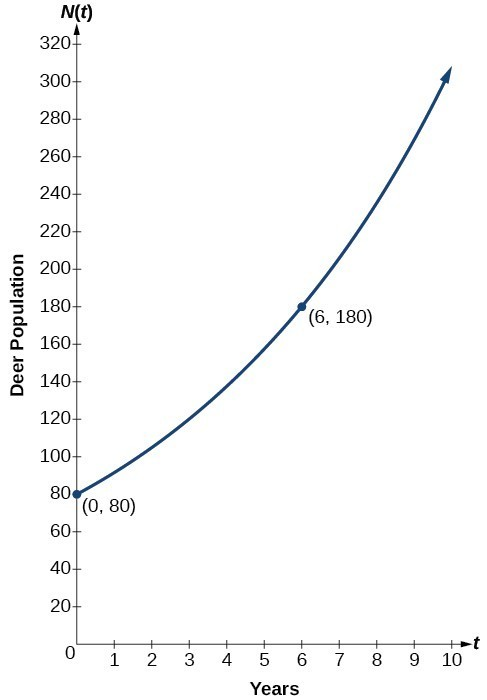Figure 3.  Graph showing the population of deer over time, $N\left(t\right)=80{\left(1.1447\right)}^{t}$, t  years after 2006

A wolf population is growing exponentially. In 2011, 129 wolves were counted. By 2013 the population had reached 236 wolves. What two points can be used to derive an exponential equation modeling this situation? Write the equation representing the population N  of wolves over time t .

## Example 4: Writing an Exponential Model When the Initial Value is Not Known

Find an exponential function that passes through the points $\left(-2,6\right)$ and $\left(2,1\right)$.

Because we don’t have the initial value, we substitute both points into an equation of the form $f\left(x\right)=a{b}^{x}$, and then solve the system for a  and b .

• Substituting $\left(-2,6\right)$ gives $6=a{b}^{-2}$
• Substituting $\left(2,1\right)$ gives $1=a{b}^{2}$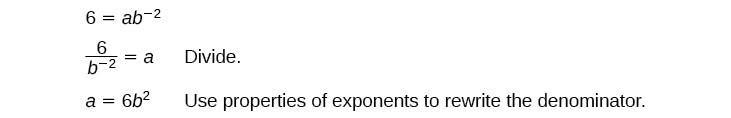Thus, the equation is $f\left(x\right)=2.4492{\left(0.6389\right)}^{x}$.

We can graph our model to check our work. Notice that the graph below passes through the initial points given in the problem, $\left(-2,\text{ 6}\right)$ and $\left(2,\text{ 1}\right)$. The graph is an example of an exponential decay function.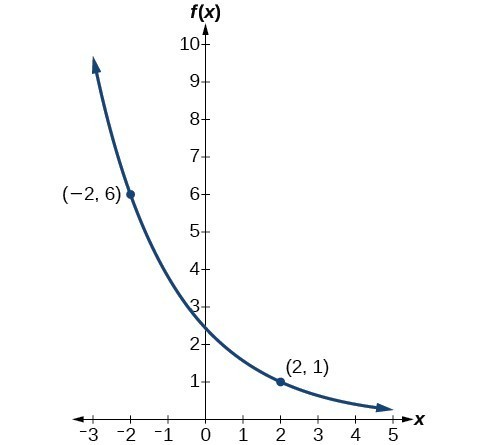Figure 4.  The graph of $f\left(x\right)=2.4492{\left(0.6389\right)}^{x}$ models exponential decay.

Given the two points $\left(1,3\right)$ and $\left(2,4.5\right)$, find the equation of the exponential function that passes through these two points.

Do two points always determine a unique exponential function?

Yes, provided the two points are either both above the x-axis or both below the x-axis and have different x-coordinates. But keep in mind that we also need to know that the graph is, in fact, an exponential function. Not every graph that looks exponential really is exponential. We need to know the graph is based on a model that shows the same percent growth with each unit increase in x , which in many real world cases involves time.

## How To: Given the graph of an exponential function, write its equation.

• First, identify two points on the graph. Choose the y -intercept as one of the two points whenever possible. Try to choose points that are as far apart as possible to reduce round-off error.
• If one of the data points is the y- intercept $\left(0,a\right)$ , then a  is the initial value. Using a , substitute the second point into the equation $f\left(x\right)=a{\left(b\right)}^{x}$, and solve for b .
• Write the exponential function, $f\left(x\right)=a{\left(b\right)}^{x}$.

## Example 5: Writing an Exponential Function Given Its Graph

Find an equation for the exponential function graphed in Figure 5.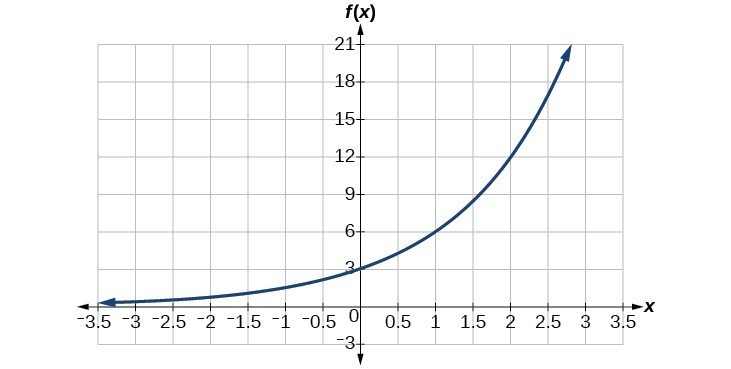We can choose the y -intercept of the graph, $\left(0,3\right)$, as our first point. This gives us the initial value, $a=3$. Next, choose a point on the curve some distance away from $\left(0,3\right)$ that has integer coordinates. One such point is $\left(2,12\right)$.

Because we restrict ourselves to positive values of b , we will use b  = 2. Substitute a  and b  into the standard form to yield the equation $f\left(x\right)=3{\left(2\right)}^{x}$.

Find an equation for the exponential function graphed in Figure 6.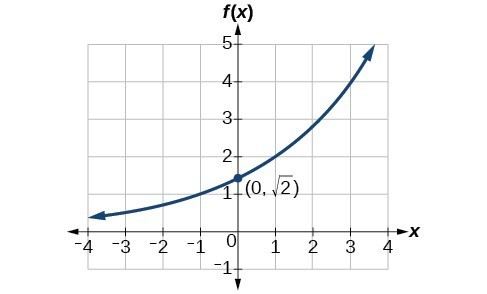## How To: Given two points on the curve of an exponential function, use a graphing calculator to find the equation.

• Press [STAT].
• Clear any existing entries in columns L1 or L2.
• In L1 , enter the x -coordinates given.
• In L2 , enter the corresponding y -coordinates.
• Press [STAT] again. Cursor right to CALC , scroll down to ExpReg (Exponential Regression) , and press [ENTER].
• The screen displays the values of a and b in the exponential equation $y=a\cdot {b}^{x}$.

## Solver Title## Generating PDF...

• Pre Algebra Order of Operations Factors & Primes Fractions Long Arithmetic Decimals Exponents & Radicals Ratios & Proportions Percent Modulo Mean, Median & Mode Scientific Notation Arithmetics
• Algebra Equations Inequalities System of Equations System of Inequalities Basic Operations Algebraic Properties Partial Fractions Polynomials Rational Expressions Sequences Power Sums Interval Notation Pi (Product) Notation Induction Logical Sets Word Problems
• Pre Calculus Equations Inequalities Simultaneous Equations System of Inequalities Polynomials Rationales Complex Numbers Polar/Cartesian Functions Arithmetic & Comp. Coordinate Geometry Plane Geometry Solid Geometry Conic Sections Trigonometry
• Calculus Derivatives Derivative Applications Limits Integrals Integral Applications Integral Approximation Series ODE Multivariable Calculus Laplace Transform Taylor/Maclaurin Series Fourier Series Fourier Transform
• Functions Line Equations Functions Arithmetic & Comp. Conic Sections Transformation
• Linear Algebra Matrices Vectors
• Trigonometry Identities Proving Identities Trig Equations Trig Inequalities Evaluate Functions Simplify
• Statistics Mean Geometric Mean Quadratic Mean Average Median Mode Order Minimum Maximum Probability Mid-Range Range Standard Deviation Variance Lower Quartile Upper Quartile Interquartile Range Midhinge Standard Normal Distribution
• Physics Mechanics
• Chemistry Chemical Reactions Chemical Properties
• Finance Simple Interest Compound Interest Present Value Future Value
• Economics Point of Diminishing Return
• Conversions Radical to Exponent Exponent to Radical To Fraction To Decimal To Mixed Number To Improper Fraction Radians to Degrees Degrees to Radians Hexadecimal Scientific Notation Distance Weight Time
• Pre Algebra
• One-Step Subtraction
• One-Step Multiplication
• One-Step Division
• One-Step Decimals
• Two-Step Integers
• Two-Step Multiply/Divide
• Two-Step Fractions
• Two-Step Decimals
• Multi-Step Integers
• Multi-Step with Parentheses
• Multi-Step Rational
• Multi-Step Fractions
• Multi-Step Decimals
• Solve by Factoring
• Completing the Square
• Logarithmic
• Exponential
• Rational Roots
• Floor/Ceiling
• Equation Given Roots
• Newton Raphson
• Substitution
• Elimination
• Cramer's Rule
• Gaussian Elimination
• System of Inequalities
• Perfect Squares
• Difference of Squares
• Difference of Cubes
• Sum of Cubes
• Polynomials
• Distributive Property
• FOIL method
• Perfect Cubes
• Binomial Expansion
• Negative Rule
• Product Rule
• Quotient Rule
• Expand Power Rule
• Fraction Exponent
• Exponent Rules
• Exponential Form
• Logarithmic Form
• Absolute Value
• Rational Number
• Powers of i
• Partial Fractions
• Is Polynomial
• Standard Form
• Complete the Square
• Synthetic Division
• Linear Factors
• Rationalize Denominator
• Rationalize Numerator
• Identify Type
• Convergence
• Interval Notation
• Pi (Product) Notation
• Boolean Algebra
• Truth Table
• Mutual Exclusive
• Cardinality
• Caretesian Product
• Age Problems
• Distance Problems
• Cost Problems
• Investment Problems
• Number Problems
• Percent Problems
• Multiplication/Division
• Dice Problems
• Coin Problems
• Card Problems
• Pre Calculus
• Linear Algebra
• Trigonometry
• Conversions## Most Used Actions

Number line.

• 8^{x-2}=\sqrt{8}
• 10^{1-x}=10^4
• 6^{3x}=2^{2x-3}
• 3^x=9^{x+5}
• What is an exponential equation?
• An exponential equation is an equation that contains an exponential expression of the form b^x, where b is a constant (called the base) and x is a variable.
• How do you solve exponential equations?
• To solve an exponential equation start by isolating the exponential expression on one side of the equation. Then, take the logarithm of both sides of the equation to convert the exponential equation into a logarithmic equation. The logarithm must have the same base as the exponential expression in the equation. Use logarithmic properties to simplify the logarithmic equation, and solve for the variable by isolating it on one side of the equation.
• What is an exponent?
• An exponent is a mathematical notation indicating the number of times a value is multiplied by itself.
• what is e in math?
• In mathematics, 'e' refers to the mathematical constant approximately equal to 2.71828. It is widely used in various mathematical and scientific fields, such as exponential and logarithmic functions.
• Is log the inverse of exponential?
• Logarithm and exponential are inverse functions. If y = b^x, where b is the base of the exponential, then x = logb(y).

exponential-equation-calculator

• High School Math Solutions – Exponential Equation Calculator Solving exponential equations is pretty straightforward; there are basically two techniques: <ul> If the exponents... Read More
• EXPLORE Tech Help Pro About Us Random Article Quizzes Request a New Article Community Dashboard This Or That Game Popular Categories Arts and Entertainment Artwork Books Movies Computers and Electronics Computers Phone Skills Technology Hacks Health Men's Health Mental Health Women's Health Relationships Dating Love Relationship Issues Hobbies and Crafts Crafts Drawing Games Education & Communication Communication Skills Personal Development Studying Personal Care and Style Fashion Hair Care Personal Hygiene Youth Personal Care School Stuff Dating All Categories Arts and Entertainment Finance and Business Home and Garden Relationship Quizzes Cars & Other Vehicles Food and Entertaining Personal Care and Style Sports and Fitness Computers and Electronics Health Pets and Animals Travel Education & Communication Hobbies and Crafts Philosophy and Religion Work World Family Life Holidays and Traditions Relationships Youth
• Browse Articles
• Learn Something New
• Quizzes Hot
• This Or That Game New
• Explore More
• Support wikiHow
• Education and Communications
• Mathematics
• Exponents and Logarithms

## How to Solve Algebraic Problems With Exponents

Last Updated: July 6, 2023 Fact Checked

In algebra, the operations (adding, subtracting, multiplying, and dividing) performed on variables work the same as the operations performed on numbers. When performing these operations on exponents, however, the laws are different. By learning these special rules for exponents, you can easily simplify algebraic expressions that include them.

## Solving a Problem with Exponents## Understanding the Laws of Exponents## Community Q&A## Video . By using this service, some information may be shared with YouTube.## Things You'll Need

You might also like.• ↑ https://www.mathsisfun.com/operation-order-pemdas.html
• ↑ https://content.nroc.org/DevelopmentalMath/COURSE_TEXT2_RESOURCE/U11_L1_T2_text_final.html
• ↑ https://www.mathsisfun.com/exponent.html
• ↑ http://www.mathwarehouse.com/algebra/exponents/index.php
• ↑ http://www.mathwarehouse.com/algebra/exponents/laws-of-exponents.php
• ↑ http://www.mathsisfun.com/algebra/negative-exponents.html• Send fan mail to authorsOct 30, 2017Samantha Brizuela

Nov 17, 2018Sammy Norman

Oct 5, 2016Crystal Ellisor-Carrier

Nov 3, 2021## Featured Articles## Trending Articles## Watch Articles• Do Not Sell or Share My Info
• Not Selling Info

wikiHow Tech Help Pro:

• + ACCUPLACER Mathematics
• + ACT Mathematics
• + AFOQT Mathematics
• + ALEKS Tests
• + ASVAB Mathematics
• + ATI TEAS Math Tests
• + Common Core Math
• + DAT Math Tests
• + FSA Tests
• + FTCE Math
• + GED Mathematics
• + Georgia Milestones Assessment
• + GRE Quantitative Reasoning
• + HiSET Math Exam
• + HSPT Math
• + ISEE Mathematics
• + PARCC Tests
• + Praxis Math
• + PSAT Math Tests
• + PSSA Tests
• + SAT Math Tests
• + SBAC Tests
• + SIFT Math
• + SSAT Math Tests
• + STAAR Tests
• + TABE Tests
• + TASC Math
• + TSI Mathematics
• + ACT Math Worksheets
• + Accuplacer Math Worksheets
• + AFOQT Math Worksheets
• + ALEKS Math Worksheets
• + ASVAB Math Worksheets
• + ATI TEAS 6 Math Worksheets
• + FTCE General Math Worksheets
• + GED Math Worksheets
• + 3rd Grade Mathematics Worksheets
• + 4th Grade Mathematics Worksheets
• + 5th Grade Mathematics Worksheets
• + 6th Grade Math Worksheets
• + 7th Grade Mathematics Worksheets
• + 8th Grade Mathematics Worksheets
• + 9th Grade Math Worksheets
• + HiSET Math Worksheets
• + HSPT Math Worksheets
• + ISEE Middle-Level Math Worksheets
• + PERT Math Worksheets
• + Praxis Math Worksheets
• + PSAT Math Worksheets
• + SAT Math Worksheets
• + SIFT Math Worksheets
• + SSAT Middle Level Math Worksheets
• + 7th Grade STAAR Math Worksheets
• + 8th Grade STAAR Math Worksheets
• + THEA Math Worksheets
• + TABE Math Worksheets
• + TASC Math Worksheets
• + TSI Math Worksheets
• + AFOQT Math Course
• + ALEKS Math Course
• + ASVAB Math Course
• + ATI TEAS 6 Math Course
• + CHSPE Math Course
• + FTCE General Knowledge Course
• + GED Math Course
• + HiSET Math Course
• + HSPT Math Course
• + ISEE Upper Level Math Course
• + SHSAT Math Course
• + SSAT Upper-Level Math Course
• + PERT Math Course
• + Praxis Core Math Course
• + SIFT Math Course
• + 8th Grade STAAR Math Course
• + TABE Math Course
• + TASC Math Course
• + TSI Math Course
• + Number Properties Puzzles
• + Algebra Puzzles
• + Geometry Puzzles
• + Intelligent Math Puzzles
• + Ratio, Proportion & Percentages Puzzles
• + Other Math Puzzles

## How to Solve Exponential Equations?

An exponential equation is an equation with exponents in which exponents (or) is part of the exponents is variable. Here, you learn more about solving exponential equations problems.When the power is variable and if it is part of an equation, it is called an exponential equation . It may be necessary to use the relationship between power and logarithm to solve the exponential equations.

## Related Topics

• How to Solve Rational Exponents
• How to Solve Rational Exponents and Radicals

## Step-by-step guide to exponential equations

There are three types of exponential equations. They are as follows:

• Equations with the same bases on both sides
• Equations with different bases can be made the same
• Equations with different bases that cannot be made the same

## Exponential equations formulas

When solving an exponential equation, the bases of both sides may be the same or may not be the same. Here are the formulas used in each of these cases.

## Solving exponential equations with the same bases

Sometimes, even though the exponents of both sides are not the same, they can be made the same. To solve exponential equations in each of these cases, we use only the property of equality of exponential equations, by which we equalize the exponentials and solve for the variable.

## Solving exponential equations with different bases

Sometimes the bases on either side of an exponential equation may not be the same (or) cannot be made the same. We solve exponential equations using logarithms when the bases on both sides of the equation are not the same. In such cases, we can do one of the following:

• Convert the exponential equation into the logarithmic form using the formula $$b^x=a⇔log _b\left(a\right)=x$$
• Apply $$log$$ on both sides of the equation and solve for the variable. In this case, we have to use the logarithm property.

## Exponential Equations – Example 1:

solve the equation $$7^x=3$$.

The bases on both sides of the exponential equation are not the same, so must apply $$log$$ on both sides of the exponential equation:

$$log 7^x=log 3$$

Then, use the property of $$log$$: $$log a^m=m \:log a$$

$$x log 7=log 3$$

Now, dividing both sides by $$log 7$$:

$$x=\frac{log 3}{log 7}$$

## Exponential Equations – Example 2:

Solve the equation $$4^{2x-1}=64$$.

The bases are not the same, but we can rewrite $$64$$ as a base of $$4$$ → $$4^3=64$$

Then, rewrite the equation as:

$$4^{2x-1}=4^3$$

With the property of exponential functions, if the bases are the same, the exponents must be equal:

$$2x-1=3 → 2x=3+1 → 2x=4$$

Now, divide each side by $$2$$:

## Exercises for Exponential Equations

Solve exponential equations..

• $$\color{blue}{\frac{81}{3^{-x}}=3^6}$$
• $$\color{blue}{5^{3x-2}=125^{2x}}$$
• $$\color{blue}{5^{2x}=21}$$
• $$\color{blue}{4^{x-2}=0.125}$$
• $$\color{blue}{3^{^{2x+1}}=15}$$
• $$\color{blue}{x=2}$$
• $$\color{blue}{x=-\frac{2}{3}}$$
• $$\color{blue}{x=\frac{log\:21}{2\:log\:5}}$$
• $$\color{blue}{x=\frac{1}{2}}$$
• $$\color{blue}{x=\frac{log\:5}{2\:log\:3}}$$

by: Effortless Math Team about 2 years ago (category: Articles )

## Effortless Math Team

• Top 10 Tips You MUST Know to Retake the AFOQT Math
• 7th Grade SOL Math Worksheets: FREE & Printable
• The Ultimate 6th Grade CMAS Math Course (+FREE Worksheets)
• Full-Length SSAT Lower Level Practice Test-Answers and Explanations
• 4th Grade SOL Math Worksheets: FREE & Printable
• What is the Best Scientific Calculator for College Students?
• The Ultimate 6th Grade RICAS Math Course (+FREE Worksheets)
• DAT Math Formulas
• How to Solve Word Problems of Elapsed Time
• The Ultimate 7th Grade SBAC Math Course (+FREE Worksheets)

## What people say about "How to Solve Exponential Equations? - Effortless Math: We Help Students Learn to LOVE Mathematics"?

No one replied yet.

## College Mathematics Practice Workbook The Most Comprehensive Review of College Math

Adult algebra for beginners the ultimate step by step guide and a comprehensive adult algebra refresher, college mathematics study guide step-by-step guide to preparing for college math, college mathematics full study guide comprehensive review + practice tests + online resources, college mathematics exercise book a comprehensive workbook + college math practice tests, college mathematics in 10 days the most effective college math crash course, pre-calculus for beginners the ultimate step by step guide to acing precalculus, college mathematics placement for beginners the ultimate step by step guide to acing college math placement test, college mathematics for beginners the ultimate step by step guide to acing college mathematics, trigonometry practice workbook the most comprehensive review of trigonometry, college mathematics prep the ultimate guide to college math + 2 practice tests, adult math workbook a comprehensive review + 2 full length adult math practice tests, pre-algebra practice workbook the most comprehensive review of pre-algebra, pre-algebra exercise book a comprehensive workbook + prealgebra practice tests, pre-algebra in 10 days the most effective pre-algebra crash course.

• ATI TEAS 6 Math
• ISEE Upper Level Math
• SSAT Upper-Level Math
• Praxis Core Math

Limited time only!

Save Over 30 %

It was $16.99 now it is$11.99

## Login and use all of our services.

Effortless Math services are waiting for you. login faster!

## Register Fast!

• Math Worksheets
• Math Courses
• Math Topics
• Math Puzzles
• Math eBooks
• GED Math Books
• HiSET Math Books
• ACT Math Books
• ISEE Math Books
• ACCUPLACER Books
• Apple Store

Effortless Math provides unofficial test prep products for a variety of tests and exams. All trademarks are property of their respective trademark owners.

• Bulk Orders
• Refund Policy

## OpenAlgebra.com

• Videos & Interactives
• Algebra Worksheets

## Solving Exponential Equations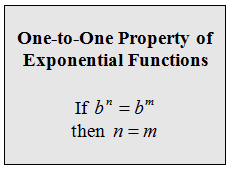#### IMAGES

1. Learn How to Solve Exponential Equations Using Two Different Methods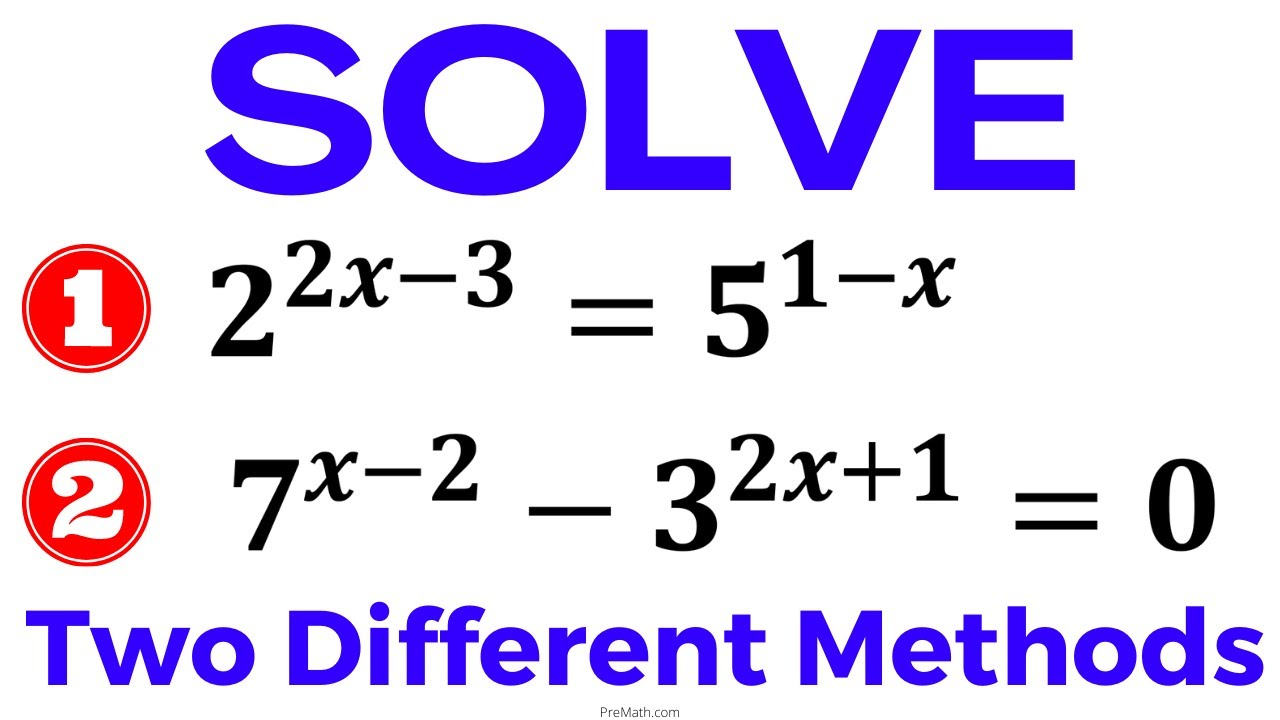2. How to solve exponential equation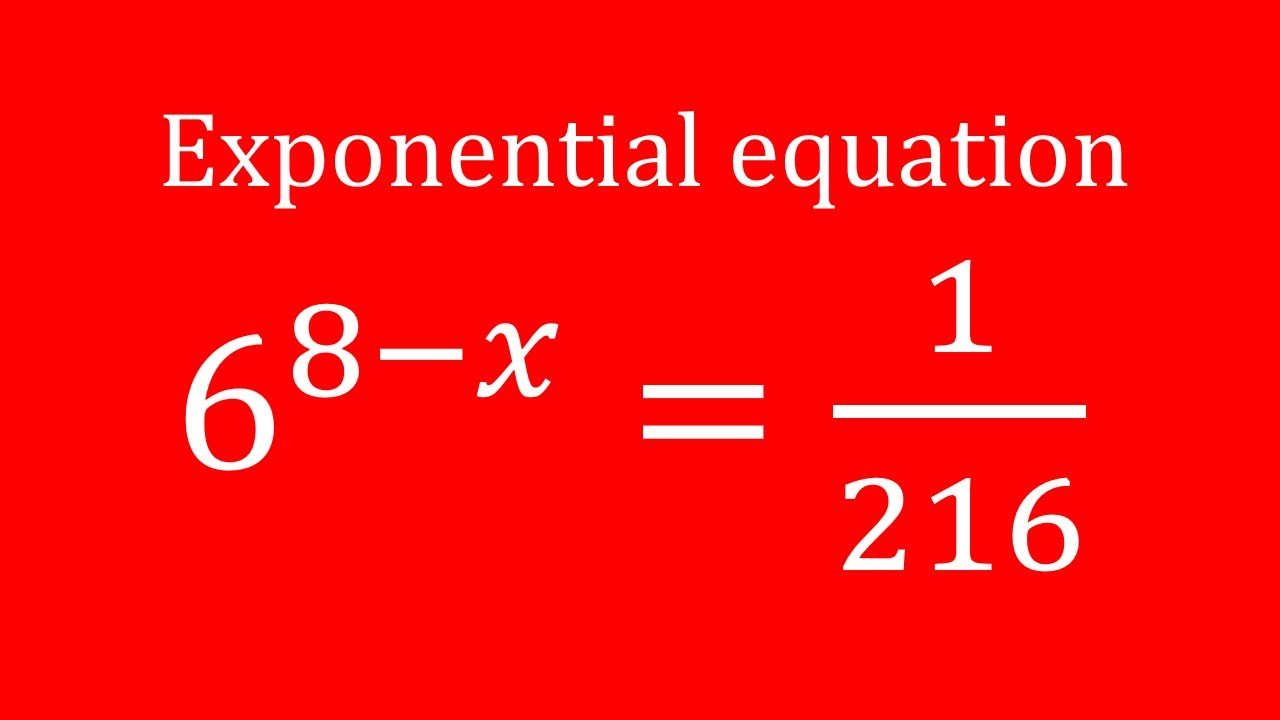3. Solve Exponential Equations X Base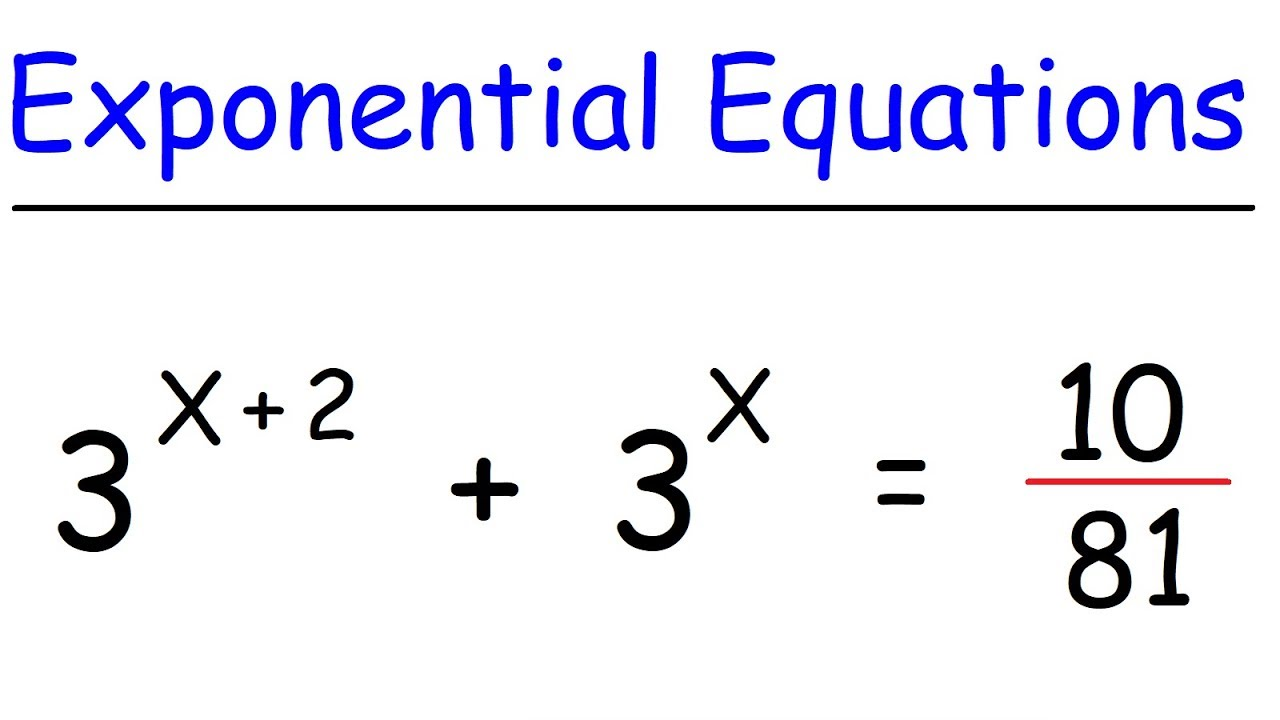4. How to Solve Algebraic Problems With Exponents: 8 Steps5. Solve Each Exponential Equation. Two Different Methods. e^(-2x) = 1/3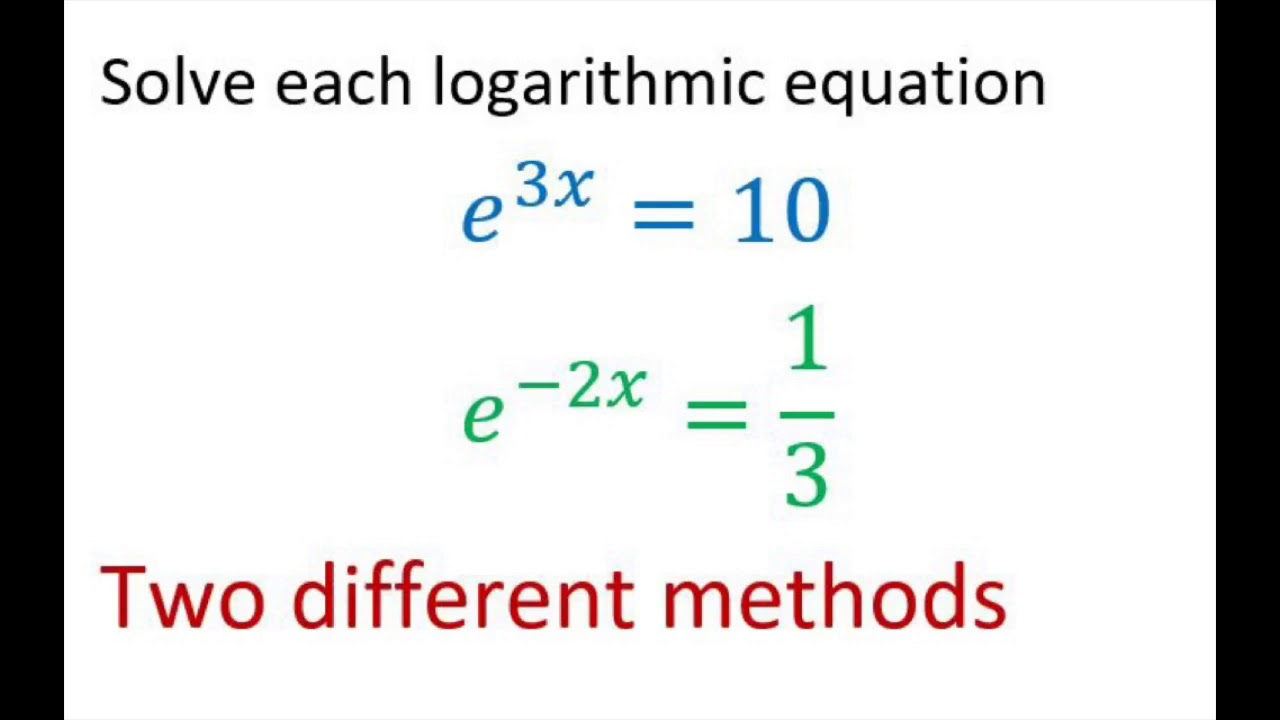6. Solving Exponential Equations (Day 3)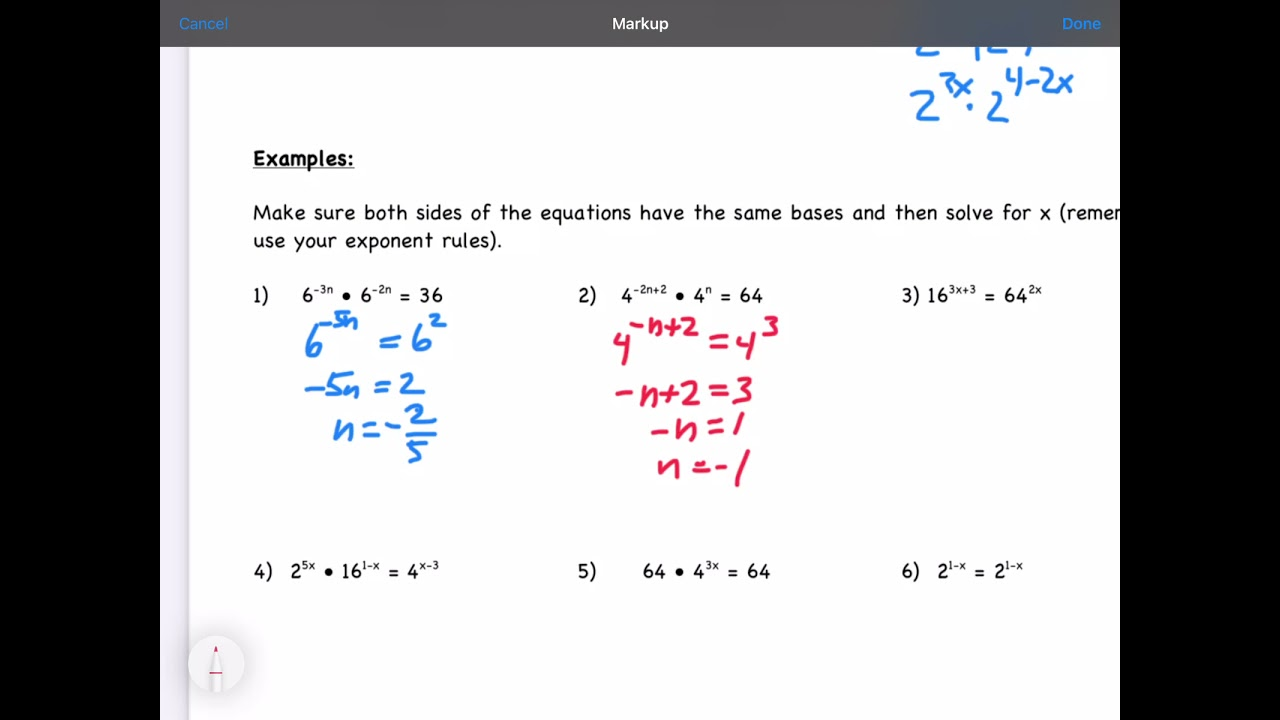#### VIDEO

1. Solving Exponential Equation

2. Solving Exponential Equation

3. A Nice Exponential Equation • X=?

4. A Nice Exponential Equation

5. A Nice Exponential Calculation/you Can Apply This Trick

6. An Algebraic Expression

1. Solving exponential equations using exponent properties

Solving exponential equations using exponent properties CCSS.Math: HSA.SSE.B.3 , HSN.RN.A.2 , HSN.RN.A Google Classroom About Transcript Sal solves equations like 26^ (9x+5) = 1 and 2^ (3x+5) = 64^ (x-7). Questions Tips & Thanks Want to join the conversation? Sort by: Top Voted letzkusb000 5 years ago

2. 3 Ways to Solve Exponential Equations

Method 1 Equating Two Exponents with the Same Base Download Article 1 Determine whether the two exponents have the same base. The base is the big number in an exponential expression.  You can only use this method when you are presented with an equation that has an exponent on either side, and each exponent has the same base. For example,

3. How to solve exponential equations

Step 1 Ignore the bases, and simply set the exponents equal to each other x + 1 = 9 Step 2 Solve for the variable x = 9 − 1 x = 8 Check We can verify that our answer is correct by substituting our value back into the original equation . . 4 x + 1 = 4 9 4 8 + 1 = 4 9 4 9 = 4 9 Exponential Equation Solver

4. Algebra

There are two methods for solving exponential equations. One method is fairly simple but requires a very special form of the exponential equation. The other will work on more complicated exponential equations but can be a little messy at times. Let's start off by looking at the simpler method.

5. Exponential Equations

a x = a y ⇔ x = y. Exponential Equations to Logarithmic Form We know that logarithms are nothing but exponents and vice versa. Hence an exponential equation can be converted into a logarithmic function. This helps in the process of solving an exponential equation with different bases.

6. Exponential Equations

How To: Given an exponential equation Of the form bS =bT b S = b T, where S and T are algebraic expressions with an unknown, solve for the unknown Use the rules of exponents to simplify, if necessary, so that the resulting equation has the form bS = bT b S = b T. Use the one-to-one property to set the exponents equal to each other.

7. Solving exponential equations using logarithms

Solving exponential equations of the form a ⋅ b x = d Let's solve 5 ⋅ 2 x = 240 . To solve for x , we must first isolate the exponential part. To do this, divide both sides by 5 as shown below. We do not multiply the 5 and the 2 as this goes against the order of operations! 5 ⋅ 2 x = 240 2 x = 48

8. 6.6 Exponential and Logarithmic Equations

Learning Objectives In this section, you will: Use like bases to solve exponential equations. Use logarithms to solve exponential equations. Use the definition of a logarithm to solve logarithmic equations. Use the one-to-one property of logarithms to solve logarithmic equations.

9. Solve exponential equations using exponent properties (advanced

Course: Algebra 2 > Unit 6. Lesson 5: Solving exponential equations using properties of exponents. Solving exponential equations using exponent properties. Solve exponential equations using exponent properties. Solving exponential equations using exponent properties (advanced)

10. Intro to exponential functions

An exponential function represents the relationship between an input and output, where we use repeated multiplication on an initial value to get the output for any given input. Exponential functions can grow or decay very quickly.

11. Exponential & logarithmic functions

Test your understanding of Exponential & logarithmic functions with these % (num)s questions. This topic covers: - Radicals & rational exponents - Graphs & end behavior of exponential functions - Manipulating exponential expressions using exponent properties - Exponential growth & decay - Modeling with exponential functions - Solving ...

12. Study Guide

How To: Given an exponential equation with the form {b}^ {S}= {b}^ {T} bS = bT, where S and T are algebraic expressions with an unknown, solve for the unknown. Use the rules of exponents to simplify, if necessary, so that the resulting equation has the form. b S = b T. {b}^ {S}= {b}^ {T} bS = bT. Use the one-to-one property to set the exponents ...

13. Solving Exponential Equations

To solve exponential equations, we need to consider the rule of exponents. These rules help us a lot in solving these type of equations. In solving exponential equations, the following theorem is often useful: Here is how to solve exponential equations: Manage the equation using the rule of exponents and some handy theorems in algebra. Use the theorem above that we just proved. If the bases ...

14. Find the equation of an exponential function

Use the first equation to solve for a in terms of b: Substitute a in the second equation, and solve for b: Use the value of b in the first equation to solve for the value of a: Thus, the equation is $f\left(x\right)=2.4492{\left(0.6389\right)}^{x}$. We can graph our model to check our work.

15. Exponential Equation Calculator

How do you solve exponential equations? To solve an exponential equation start by isolating the exponential expression on one side of the equation. Then, take the logarithm of both sides of the equation to convert the exponential equation into a logarithmic equation.

16. How to Solve Algebraic Problems With Exponents: 8 Steps

Method 1 Solving a Problem with Exponents Download Article 1 Address the order of operations. Just like any problem in mathematics, an algebraic problem must be completed by the order of operations.

17. How to Solve Exponential Equations?

Exponential equations formulas. When solving an exponential equation, the bases of both sides may be the same or may not be the same. Here are the formulas used in each of these cases. Solving exponential equations with the same bases. Sometimes, even though the exponents of both sides are not the same, they can be made the same.

18. 4.7: Exponential and Logarithmic Equations

Use the rules of exponents to simplify, if necessary, so that the resulting equation has the form bS = bT. Use the one-to-one property to set the exponents equal. Solve the resulting equation, S = T, for the unknown. Example 4.7.1: Solving an Exponential Equation with a Common Base. Solve 2x − 1 = 22x − 4.

19. Solving Exponential Equations

This algebra video tutorial explains how to solve exponential equations using basic properties of logarithms and natural logs. Examples include factoring tr...

20. Exponential Equations

This Algebra and Precalculus video tutorial explains how to solve difficult exponential equations.Algebra For Beginners: ...

21. Algebra

Here is a set of practice problems to accompany the Solving Exponential Equations section of the Exponential and Logarithm Functions chapter of the notes for Paul Dawkins Algebra course at Lamar University.

22. Solving Exponential Equations

Step 1: Isolate the exponential and then apply the logarithm to both sides. Step 2: Apply the power rule for logarithms and write the exponent as a factor of the base. Step 3: Solve the resulting equation. Solve.

23. How to Solve Exponential Equations

Exponential equations can have any positive integer as the base number except for one . One raised to any power is just one. Here are two examples that have the same base number: y = 4 x − 5 and ...

24. How To Solve Exponential Equations Mathematics

How To Solve Exponential Equations Mathematics - Maths - Algebra #maths #mathematics #shorts

25. Algebra 2 Worksheet Solving Exponential Equations

Guest 1/7 algebra-2-worksheet-solving-exponential-equations Algebra 2 Worksheet Solving Exponential Equations Algebra 1 2014-07-22 This student-friendly, all-in-one workbook contains a place to work through Explorations as well as extra practice workskeets, a glossary, and manipulatives. The Student Journal is available in Spanish in both print ...